Courses

# Math Formulas For GMAT GMAT Notes | EduRev

## Quantitative Aptitude for GMAT

Created by: Wizius Careers

## GMAT : Math Formulas For GMAT GMAT Notes | EduRev

The document Math Formulas For GMAT GMAT Notes | EduRev is a part of the GMAT Course Quantitative Aptitude for GMAT.
All you need of GMAT at this link: GMAT

MATH FORMULAS

The most powerful EMPOWER tactic for the Quantitative section of the GMAT

WHY Learn GMAT Math Formulas?

1. Including Geometry, more than half the questions in the Quant section involve a speciﬁc math formula (or more than 1), so knowing the formulas is a must to solving the problem.
2. Math formulas can be used to answer many different versions of the same question.
3. TRIAGE can become easier in some cases by using the formula to estimate the approximate answer to a question.

Area & Perimeter Formulas
Square:

• Area = length2
• Perimeter = 4*length

Rectangle:

• Area = length*width
• Perimeter = 2(length) + 2(width)

Parallelogram:

• Area = base*height
• Perimeter = 2(base) + 2(height)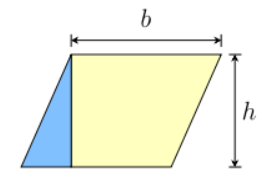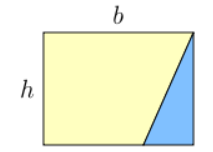Circles:

• Area = πr2
• Circumference = 2πr

Triangle:

•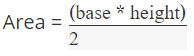• Pythagorean Theorem: a2+b2=c2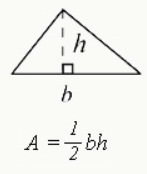Trapezoid:

• Area =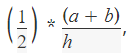where a and b are the length of the parallel sides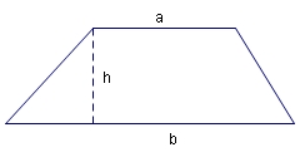Circle Formulas
Central angle = 2*(inscribed angle)
Area of a sector =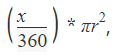where x is the measurement of the central angle of the circle portion in degrees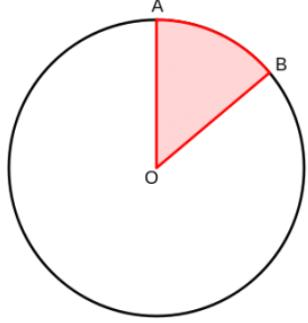Volume formulas
Cube: (length)3
Cylinder: πr2*h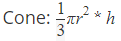Pyramid: 1/3 (base length*base width*height)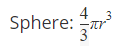GMAT Arithmetic Formulas
Arithmetic concepts are one of the most heavily tested content areas on the GMAT quant section, making up nearly 50% of GMAT quant question types. These GMAT quant formulas address the majority of concepts covered on the GMAT.

Order of operations
Parentheses – Exponents – Multiplication – Division – Addition – Subtraction (PEMDAS)

Number properties
(Positive Number) * (Positive Number) = (Positive Number)
(Positive Number) * (Negative Number) = (Negative Number)
(Negative Number) * (Negative Number) = (Positive Number)
(Positive Number) / (Positive Number) = (Positive Number)
(Positive Number) / (Negative Number) = (Negative Number)
(Negative Number) / (Negative Number) = (Positive Number)
(Odd Number) + (Odd Number) = (Even Number)
(Odd Number) – (Odd Number) = (Even Number)
(Odd Number) + (Even Number) = (Odd Number)
(Odd Number) – (Even Number) = (Odd Number)
(Even Number) + (Even Number) = (Even Number)
(Even Number) – (Even Number) = (Even Number)
(Odd Number) * (Odd Number) = (Odd Number)
(Odd Number) * (Even Number) = (Even Number)
(Even Number) * (Even Number) = (Even Number)Permutations and Combinations

Permutation formula: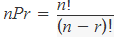Combination formula: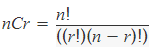Probability
Probability = (Number of favorable outcomes) / (Number of all possible outcomes)

Probability of events A & B happening = (Probability of A) * (Probability of B)
Probability of either event A or B happening = (Probability of A) + (Probability of B)

GMAT Algebra Formulas
Algebra questions make up about 20% of the question types you’ll see on the GMAT quant section.

Absolute Value
|x| depicts absolute value.
|x| = x
|-x| = x
|x| = |-x|
|x| ≥ 0
|x| + |y| ≥ |x+y|

Exponent Rules
In the expression xn, ‘x’ is the base and ‘n’ is the exponent. The way to interpret is that the base ‘x’ gets multiplied ‘n’ times. For example, 23=2*2*2.
Some key rules and formulas for exponents:
0n=0
1n=1
x0=1

x1=x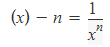xm*xn=x(m+n)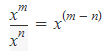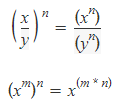ax2+bx+c=0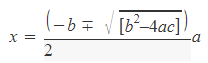Interest
All interest formulas use the following variables: P = starting principle; r = annual interest rate; t = number of years.
Simple Interest = P*r*t
Annual Compound Interest = P(1+r)t
Compound Interest = P(1 + r/x)^(xt); x = number of times the interest compounds over the year

Other Algebra Formulas
Distance = Speed * Time
Wage = Rate * Time

Offer running on EduRev: Apply code STAYHOME200 to get INR 200 off on our premium plan EduRev Infinity!

## Quantitative Aptitude for GMAT

85 videos|100 docs|176 tests

,

,

,

,

,

,

,

,

,

,

,

,

,

,

,

,

,

,

,

,

,

;